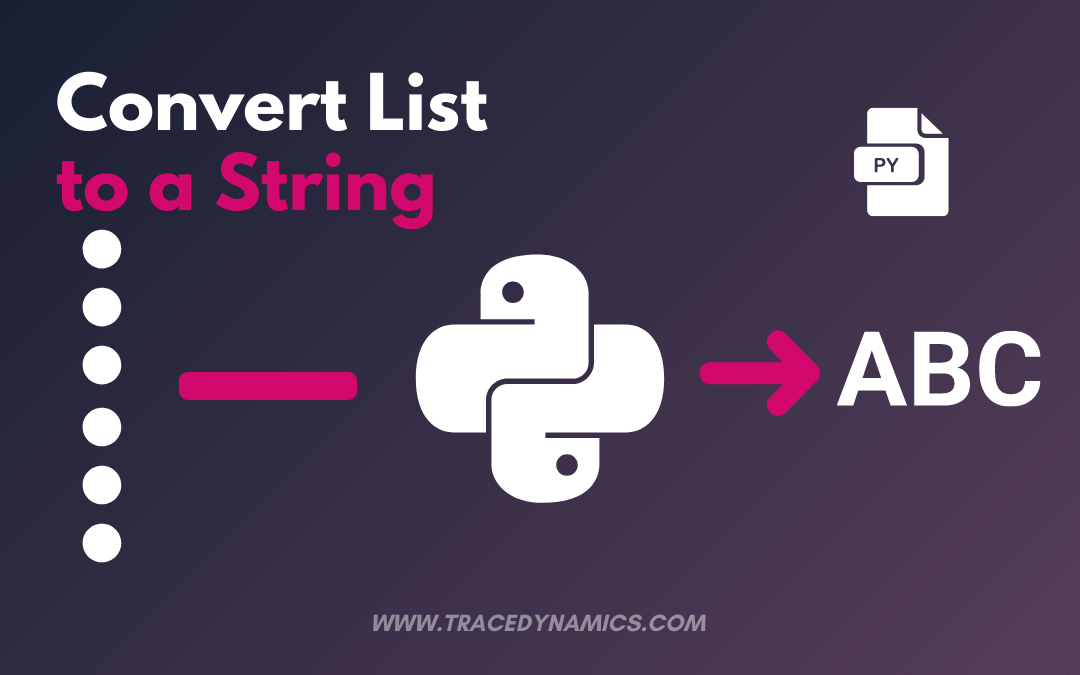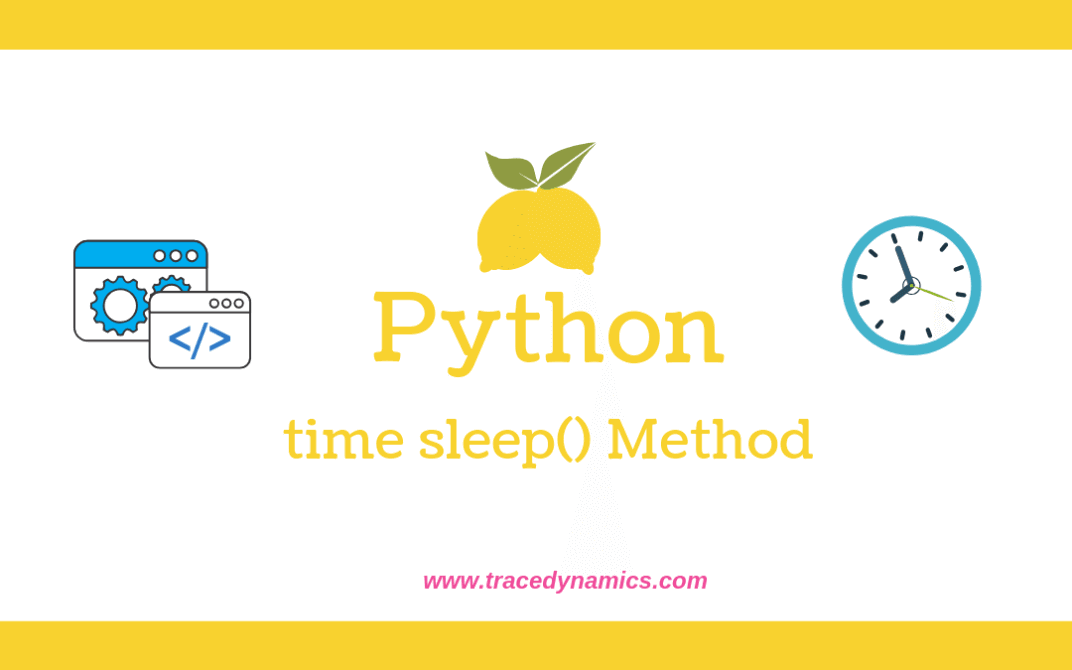## Python: Convert List to a String

Looking to convert list to string in python?In this post, we have covered about the best ways to convert list to string.You can learn and implement it very easily.

To Convert a list to a string in python, You can do by,

1. Using join()
2. Using map()
3. With List Comprehension
4. Through Iteration

## Using join()

From the below Python program, let’s see how to convert Python list to string using the join() method.

We are passing a list of array elements as input to the python join() method.

In other words, we are passing a list of integers as an array to list.

``````x = ['Welcome', 'to', 'Trace', 'Dynamics']
result = ' '.join(x)
print(result)

Output:
Welcome to Trace Dynamics
``````

## Using map()

From the below Python program, let’s see how to convert list to python string using the map() method.

We are passing a list of array elements as input to the python map() method.

In other words, we are passing a list of integers as an array to list.

``````x = ['Welcome', 'to', 'Trace', 'Dynamics']
result = ' '.join(map(str, x))
print(result)

Output:
Welcome to Trace Dynamics
``````

As per the output code above, we can see a list of array values printed as a string.

You May Like,

## List Comprehension

let’s see how to convert Python list to string using the list comprehension.

``````x = ['Welcome', 'to', 'Trace', 'Dynamics']
result =  ' '.join([str(value) for value in x])
print(result)

Output:
Welcome to Trace Dynamics
``````

## Through Iteration

let’s see how to convertlist to string using iteration in Python.

``````x = ['Welcome', 'to', 'Trace', 'Dynamics']
result = ""
for element in x:
result += element
print(result)

Output:
Welcome
Welcometo
WelcometoTrace
WelcometoTraceDynamics
``````

To conclude, in this tutorial we gone through different ways to convert a Python List to a String.

List to String methods conversion is also common in other programming languages like JavaScript, Python, jQuery.

Keeping sharing tutorials and happy coding 🙂## 6 Best Ways to Compute HCF or GCD in Python

GCD Python: In this article, we have discussed different ways of computing GCD of two numbers in Python.

Note that GCD stands for Greatest Common Divisor.

GCD also called as HCF(Highest Common Factor).

We can calculate GCD by using Recursion Function, Loops, Euclidean algorithm, Math, Fractions and Numpy libraries.

## What is GCD?

GCD is nothing but Greatest Common Divisor or Highest Common Factor of input numbers.

The Largest Integer will divide and return the difference.

For example, The GCD of 14 and 16 is 2.

## Different Ways to Compute GCD

GCD can be computed by following ways.

• Using Recursion Function
• Leveraging Loops
• Using Euclidean Algorithm
• With math.gcd() Function

### Using Recursion Function

Recursion is nothing but a mechanism in which a function calls itself.

Using recursion program, solution to the bigger problem is mentioned in terms of smaller problems.

Lets go through the implementation below.

``````def recursiveGCD(a,b):
if(b==0):
return a
else:
return recursiveGCD(b,a%b)
a=54
b=72

print("The GCD is", recursiveGCD(a, b))

Output:
The GCD is 18
``````

### Leveraging Loops

Lets compute GCD by leveraging the loop.

``````def loopGCD(a, b):

if a > b:
tiny = b
else:
tiny = a
for j in range(1, tiny+1):
if((a % j == 0) and (b % j == 0)):
finalgcd = j

return finalgcd

a=54
b=72

print("The GCD is", loopGCD(a, b))

Output:
The GCD is 18
``````

You May Also Like,

### Using Euclidean Algorithm

Mathematically Euclidean Algorithm is an impressive way for computing the greatest common divisor (GCD) of two numbers (integers), where the largest number that divides both without having a remainder.

This Algorithm is named after the ancient Greek mathematician Euclid.

Using Euclidean Algorithm, we can compute GCD by leveraging as below.

``````def algoGCD(a, b):

while(b):
a, b = b, a % b

return a

a=54
b=72

print("The GCD is", algoGCD(a, b))

Output:
The GCD is 18
``````

### With math.gcd() Function

The math package provides gcd() method , which will compute the gcd of given numbers.

Its just a one line code.

``````import math

a=54
b=72

print("The GCD is", math.gcd(a, b))

Output:
The GCD is 18
``````

### Using fractions.gcd() Function

The fractions package provides gcd() method, this will calculate the gcd with one line of code.

``````import fractions

a=54
b=72

print("The GCD is", fractions.gcd(a, b))

Output:
The GCD is 18
``````

You May Also Love,

### Using numpy.gcd() Function

The numpy package provides gcd() method, using this we can compute the gcd with one line of code.

Note that gcd() method is added into numpy library from version 1.15.

So if your using any version older than 1.15, then gcd function will not be available.

``````import numpy

a=54
b=72

print("The Value is", numpy.gcd(a,b))

Output:
The GCD is 18
``````

#### Conclusion

Above are the some of the best methods to compute GCD in Python.

To conclude this tutorial, we covered various methods with examples to calculate HCF of two numbers using Python progamming language.## Python Remove Character From String: Learn How To Remove On Python

In this article, we have discussed how can you remove the character from string in python.

Let’s discuss how to remove a specific character or unwanted character from string in this post.

The following are the different string methods to remove the character from a string element using python programming.

As String is immutable, the methods used below will return a new string and the original string remains unchanged.

In other words, we are just doing string manipulation to achieve the result.

## Python Remove Character From String

remove a character from the string in python you can do by two different methods, one is using translate function and the second one is using replace functions, You can remove by using both translate and replace functions. In this article, we have discussed all the steps and methods of how can you remove it in python. Read this article fully, and if you have any doubts don’t hesitate to ask us in the comments section. You can also rate us on the bottom-left section of the vote.

## Python Remove Character from String Using the translate method

Python translate() method replaces each character in the given string inline to the provided translation table.

for this, we have to provide the Unicode for the character and None as replacement value to remove the value from the final string.

ord() function used to retrieve the Unicode of a character.

Let’s write a Python program to remove a character in the string using a translate function.

``````inputValue = 'xyz987zyx'
result = inputValue.translate({ord(i): None for i in 'xyz'});
print(result);

Output:
987
``````

## Python Remove Character from String Using the replace method

Python replaces() method replaces character with a given replacement character.

so we can provide the replacement character as empty “” or whitespace, then the character will be removed.

Let’s write a Python program to remove the first character in the original string using replace() function.

``````inputValue = 'Tracedynamics'
result = inputValue.replace('T', '');
print(result);

Output:
racedynamics
``````

As per the output above, we can see in the new string the character ‘T’ is removed.

similarly, if you want to remove the last character, then substitute the replace method with ‘s’ character.

You May Like,

## Python Remove string number of times

replace() method accepts a third parameter or argument.

This parameter can be no of times a specified string can be replaced.

In the below program, let provide an empty string to remove the specified characters using replace() function.

``````inputValue = 'Tracedynamics'
result = inputValue.replace('a', '', 2);
print(result);

Output:
Trcedynmics
``````

## Remove newline from string

We can remove a newline from python string using replace() method in the below example.

if there is any whitespace character, we can also remove using replace() method.

``````inputValue = 'Trace\ndyna\nmics'
result = inputValue.replace('\n', '');
print(result);

Output:
Tracedynamics
``````

## Removing substring from a string

We can remove a certain part of a string(substr) using replace() method.

``````inputValue = 'Tracedynamics'
result = inputValue.replace('dynamics', '');
print(result);

Output:
Trace
``````

You May Also Love,

## Remove space from a string

Let’s remove space from a python string using replace() method in the below example.

``````inputValue = 'T r a c e'
result = inputValue.replace(' ', '');
print(result);

Output:
Trace
``````

for removing any special characters, we can use the regex pattern(regular expression) in the replace method.

To conclude this tutorial, we covered various types of implementation to remove a character from string using Python Programming..## Sleep() Python Method

sleep python method pauses/suspends the python program for certain amount of specified time.In other words, sleep method suspends the execution of the calling thread for a given number of seconds.

The method argument can be a floating point to specify a more precise sleep time.Also note that, the actual suspension time might be less than the requested time. Its because of any caught signal which will terminate the sleep() following execution.

Python time.sleep( ): time sleep method is similar to shell sleep command. It accepts the argument in seconds/milli seconds.

For precise sleep times, floating point number can be provided as the argument for python time sleep() method.

Note: time sleep method is part of time module/package in python 2 and python 3, so you need to import the “time” package in the respective python script or function.

Since python version 3.5, the sleep function now sleeps atleast seconds eventhough if the sleep is interrupted by a signal, except incase of signal handler raises an exception via script.

### Syntax of Python time.sleep( ):

Following is the syntax for sleep method

time.sleep( t )

Arguments:

t – Number of seconds/milliseconds execution to be suspended in python code.

### Example 1:

Following example program illustrates the exact usage of time sleep( ) method in the python script or function.

``````import time
# Print the start time
print("current time start: ", end ="")
print(time.ctime())

# using sleep() to pause the code execution
time.sleep(5)

# Print the end time
print("current time end: ", end ="")
print(time.ctime()) ``````

Output:

``````current time start: Wed May 29 16:50:48 2019
current time end: Wed May 29 16:50:53 2019``````

As per the program execution, the wait time or delay is 5 seconds.

### Example 2: Delay in Python script

Create a script called sleep-demo.py:

``````#!/usr/bin/python
# The program will run infinity times on screen till user hit the CTRL+C
# The program will sleep for 10 seconds before updating date and time again.

import time
print "!!! Hit CTRL+C to stop the program !!!"
## Start loop ##
while True:
### Show today's date and time ##
print "Current date & time " + time.strftime("%c")
#### Delay for 10 seconds ####
time.sleep(10)

Save, close the file and run as follows:
\$ chmod +x sleep-demo.py
\$ ./sleep-demo.py
``````

Output:

``````Current date & time Wed May 29 17:50:48 2019
Current date & time Wed May 29 17:50:58 2019
Current date & time Wed May 29 17:51:08 2019
Current date & time Wed May 29 17:51:18 2019
Current date & time Wed May 29 17:51:28 2019
Current date & time Wed May 29 17:51:38 2019``````

The output will be infinite loop till user interrupts(CTRL+C).

Python supports multithreading too, here’s an example of a multithreaded Python program.

``````#!/usr/bin/python

import time

def print_welcome():
for i in range(3):
time.sleep(0.6)
print("Welcome")

def print_python():
for i in range(3):
time.sleep(0.9)
print("Python")